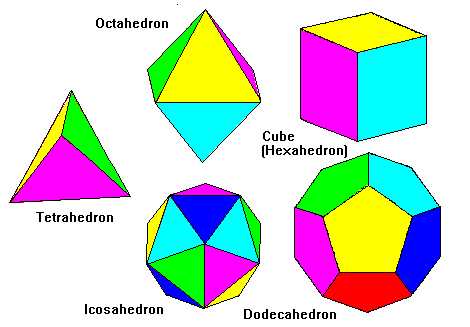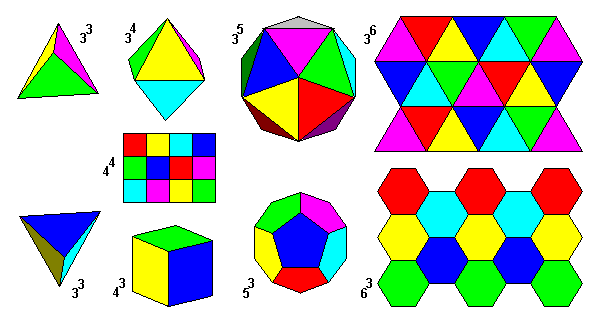# Platonic Solids

Steven Dutch, Professor Emeritus, Natural and Applied Sciences, University of Wisconsin - Green Bay

The Platonic solids are convex polyhedra with regular polygon faces. Faces and vertices are identical. There are only five of them, shown below.## How Do We Know There Are Only Five?

First of all, we need to know the vertex angles for a regular polygon of n sides. We can draw radii from the center to divide the polygon into n triangles. The total of all the angles of all the triangles is thus 180n. The angles at the center of the polygon add up, of course, to 360, so the angles around the edge of the polygon must total 180n-360 or 180(n-2). Each vertex angle, then, equals 180(n-2)/n. Thus the angle of an equilateral triangle is 60 degrees (180(3-1)/3), a square is 90 degrees (180(4-2)/4), a pentagon is 108 and a hexagon is 120.

There have to be at least three polygons at a vertex; two would simply fold together back to back. Also the angles around a vertex cannot exceed 360 degrees, and if they equal 360 degrees, the polygons simply tile the plane. Thus we can rule out solids with 6 or more faces - hexagons tile the plane and all other triplets exceed 360 degrees. That means convex solids with regular polygon faces can only have triangles, squares or pentagons as faces. The only possibilities are 3, 4, or 5 triangles, 3 squares or 3 pentagons. Thus there are only five platonic solids.

Basic Properties of Platonic Solids
Solid Faces Edges
of Face
Vertices Edges at
Vertex
Edges Dual of...
Tetrahedron 4 3 4 3 6 Tetrahedron
Cube 6 4 8 3 12 Octahedron
Octahedron 8 3 6 4 12 Cube
Dodecahedron 12 5 20 3 30 Icosahedron
Icosahedron 20 3 12 5 30 Dodecahedron

Note that in each case, Euler's Rule is followed: F + V = E + 2. Also note that faces in the cube and vertices in the octahedron play similar roles, and similarly for the dodecahedron and icosahedron. These solids are duals of each other.

## Platonic Solids and Tilings

Platonic solids and uniform tilings are closely related as shown below. Starting from the tetrahedron we have polyhedra with three, four and five triangles at each vertex, then the plane tiling with six triangles (top row). Also starting from the tetrahedron we have polyhedra with three triangles, squares and pentagons at each vertex, then the plane tiling with three hexagons (bottom row). The figures in the top and bottom rows are duals of each other; the tetrahedron is its own dual.The square tiling is placed between the cube and octahedron. We can regard it as the next step from the octahedron (four polygons at a vertex) or from the cube (three and four squares at a vertex). The square tiling is its own dual.

In addition to the convex Platonic solids, there are four other solids with identical faces and vertices. In these solids the faces penetrate through each other. These are the Kepler-Poinsot Solids.

Created 2 Oct. 1997, Last Update 18 June 1999